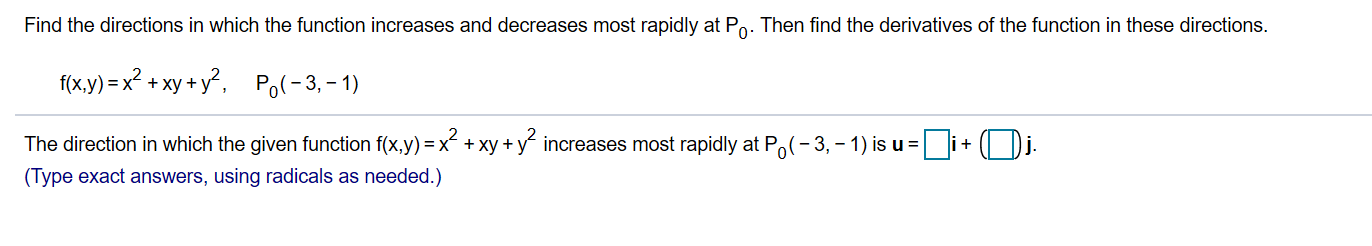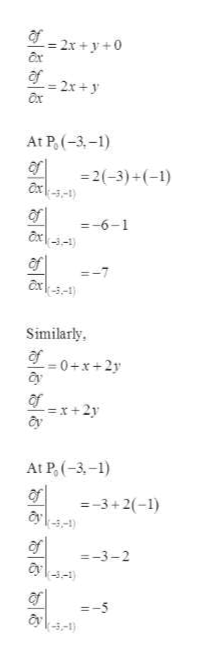# Find the directions in which the function increases and decreases most rapidly at Po. Then find the derivatives of the function in these directionsf(x.y) x2 +xy+y2Po-3,-1)The direction in which the given function f(x,y) x xy+yincreases most rapidly at Po(-3, -1) is u(Type exact answers, using radicals as needed.)

Question
15 views

Find the direction f(x,y) increases and decreases most rapidly at point P and find the derivatives of the functions in these directions (f(x,y) and P included in the image)help_outlineImage TranscriptioncloseFind the directions in which the function increases and decreases most rapidly at Po. Then find the derivatives of the function in these directions f(x.y) x2 +xy+y2 Po-3,-1) The direction in which the given function f(x,y) x xy+yincreases most rapidly at Po(-3, -1) is u (Type exact answers, using radicals as needed.) fullscreen
check_circle

Step 1

Consider the given function.

Step 2

To find the gradient of the function is the vector of partial derivatives.help_outlineImage Transcriptionclose2x+y+0 af - 2x +y Ox At P(3,-1) =2(-3)+ (-1) axk--4) of -6-1 of exk-- =-7 Similarly g0+x+2y af x+2y At P,(-3,-1) =-3+2(-1) y\ --3-2 --1) --5 -3-1) fullscreen
Step 3

Therefore, the direc...

### Want to see the full answer?

See Solution

#### Want to see this answer and more?

Solutions are written by subject experts who are available 24/7. Questions are typically answered within 1 hour.*

See Solution
*Response times may vary by subject and question.
Tagged in

### Other